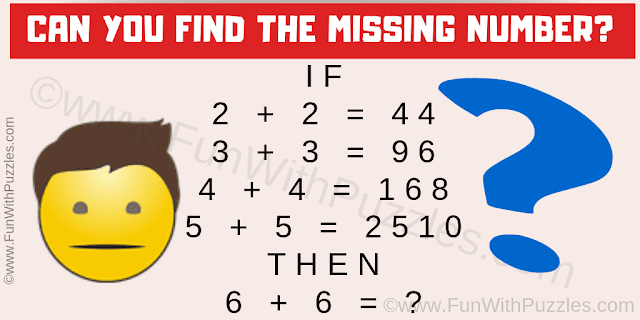This is a very interesting logic math question which will test and train your brain. Study the given logical equation and find the hidden relationship among the given numbers. Once you are able to find this logical reasoning used in these number equation, try to solve the last equation by applying the same logic. Let us see if you can find the value of the missing number which replaces the question mark!Can you find the value of the missing number?
Answer of this "Logic Math Question", can be viewed by clicking on the answer button.